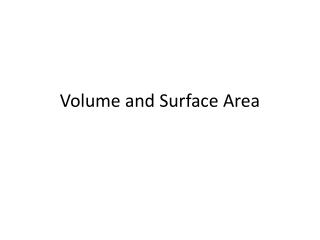DownloadDownload PresentationVolume and Surface Area

# Volume and Surface Area

Télécharger la présentation## Volume and Surface Area

- - - - - - - - - - - - - - - - - - - - - - - - - - - E N D - - - - - - - - - - - - - - - - - - - - - - - - - - -
##### Presentation Transcript

1. Volume and Surface Area

2. Circumference and area of circles • learning objectives: • find the circumference of circles and areas enclosed by circles • recall and use the formulae for the circumference of a circle and the area enclosed by a circle • use ∏≈ 3.142 or use the ∏ button on a calculator • find the perimeter and area of semicircles and quarter circles • calculate the length of arcs and the area of sectors of circles • answers in terms of ∏ may be required • find the surface area of a cylinder • Reference material • Text chapter 10 page 133 • 10 Ticks • MEP book

3. Your turn chapter 10 page 133

4. Volume and Surface Area • learning objectives: • Find the volume of a cylinder • use volume to solve problems • solve problems involving more complex shapes and solids, including segments of circles and frustums of cones • find the surface areas and volumes of compound solids constructed from cubes, cones, pyramids, spheres, hemispheres and cylinders • solve problems including examples of solids in everyday use • find the area of the segment of a circle given the radius and length of the chord • Reference Material • Text: Chapter 11 page 148 • 10 Ticks: • MEP:

5. Your turn chapter 11 page 148 10 Ticks Level 9 Pack 1 page 3-4